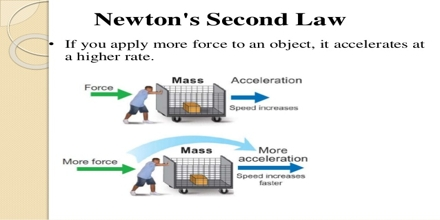Physics

# Newton’s Second Law of Motion: ExplanationIsaac Newton was the first to connect the concepts of force and mass to produce acceleration. This lecture briefly explain Newton’s Second Law of Motion. Newton’s second law (the law of acceleration) relates acceleration to force. The acceleration produced by a net force on an object is directly proportional to the net force, is in the same direction as the net force, and is inversely proportional to the mass of the object.